International Journal of Mechanical Engineering and Applications
Volume 4, Issue 6, December 2016, Pages: 232-241

Generalized Synchronization of Fractional Order Chaotic Systems with Time-Delay

Sha Wang*, Jie Li, Renhao Jin

School of Information, Beijing Wuzi University, Beijing, China

Email address: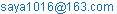(Sha Wang)

*Corresponding author

To cite this article:

Sha Wang, Jie Li, Renhao Jin. Generalized Synchronization of Fractional Order Chaotic Systems with Time-Delay. International Journal of Mechanical Engineering and Applications. Vol. 4, No. 6, 2016, pp. 232-241. doi: 10.11648/j.ijmea.20160406.14

Received: July 22, 2016; Accepted: October 27, 2016; Published: December 17, 2016

Abstract: Generalized synchronization of time-delayed fractional order chaotic systems is investigated. According to the stability theorem of linear fractional differential systems with multiple time-delays, a nonlinear fractional order controller is designed for the synchronization of systems with identical and non-identical derivative orders. Both complete synchronization and projective synchronization also can be realized based on the proposed controller. The effectiveness and robustness of the controller are verified in the numerical simulations.

Keywords: Fractional Order, Chaos, Nonlinear Control, Generalized Synchronization, Time-Delay

1. Introduction

Chaos synchronization has been a hot subject in the field of nonlinear science due to its wide-scope potential applications in physical systems, biological science, chemical reactor, etc . In 1990, Pecora and Carroll [2,3] presented complete synchronization of two identical chaotic systems with different initial conditions. The drive and response systems have the same trajectory via a suitable controller. Then, complete synchronization attracts considerable attention of the scientists [4,5]. However, it is difficult to make the drive and response systems achieve complete synchronization in the real applications. To solve this problem, Mainieri and Rehacek proposed projective synchronization in Ref. , where the drive and response systems synchronized up to a scaling factor. Its proportional feature extends binary digital to M-nary digital communication for achieving fast communication . In 1995, Rulkov et al. considered generalized synchronization, where the states of the response system synchronized with the map of the ones in the drive system . The scaling map can be arbitrary designed to the state variables. And the unpredictability of the scaling map in generalized synchronization can additionally enhance the security of communication. Both complete synchronization and projective synchronization belong to generalized synchronization. Now, many researchers studied generalized synchronization of the integer order chaotic systems in Refs. [9-12].

Fractional calculus is supposed to be a generalization of integration and differentiation of arbitrary orders . Over the last decades, the applications of fractional calculus to physics, engineering and control processing have been widely studied [14,15]. Lots of systems in interdisciplinary field can be described by the fractional differential equations, such as viscoelastic system, dielectric polarization, electrode-electrolyte polarization and financial system. With the introduction of fractional derivative, chaotic synchronization of fractional order dynamical systems becomes an active research field due to its great potential applications especially in secure communication and control processing [16-18]. For example, Si et al. discussed the projective synchronization of fractional order chaotic systems with non-identical orders . Suwat provided a feedback controller for the robust synchronization of fractional order unified chaotic systems based on the developed LMI stabilization condition . Wang et al. deliberated on the synchronization of uncertain fractional order chaotic systems with external disturbance by a fractional terminal sliding mode control . And Aghababa considered the finite-time chaos synchronization of fractional order systems based on the fractional Lyapunov stability theorem . All of these examples clarify the importance of consideration and analysis of the fractional order chaotic systems and their synchronization.

A time-delay always exists in the engineering application due to the transportation lag or the feedback delay. And the time-delayed differential models frequently apply in the physics, economics an biology [23-25]. In 1977, Macky and Glass first found chaos in the time-delayed systems . Introduction of delay in the system enriches its dynamics and allows a precise description of the real life phenomena. Then the time-delayed chaotic systems and its synchronization become a hot topic in nonlinear science [27-29]. For instance, Botmart et al. considered the synchronization of non-autonomous integer order chaotic systems with time-varying delay based on the delayed feedback control . Li et al. deliberated the lag synchronization of coupled time-delayed integer-order chaotic systems and its applications to secure communication . To the best of our knowledge, most of the existing results focused on the chaotic synchronization of time-delayed integer-order chaotic systems. There are only a few conclusions on the synchronization of time-delayed fractional order chaotic systems. In 2007, Deng et al. discussed the stability of linear fractional differential systems with multiple time-delays . In 2011, Zhou et al. advised a washout filter control for the complete synchronization of a class of fractional order neural network model with varying time-delay based on the Laplace transformation theory . In 2015, Behinfaraz et al. studied the modified projective synchronization of different fractional order chaotic systems with time-varying delays . However, some other types of synchronization for non-identical structural time-delayed fractional order chaotic systems are still open problems.

Motivated by the above discussion, the generalized synchronization of time-delayed fractional order chaotic systems is investigated in this work. Complete synchronization, anti-phase synchronization and projective synchronization are the special cases of the generalized synchronization. Both identical and different structural systems can be applied to realize the synchronization. The fractional order chaotic systems with or without time-delay also can be used for achieving the generalized synchronization. Moreover, the effect of bounded noise in the generalized synchronization is discussed in the numerical analysis.

The remainder of this letter is organized as follows. In Section II, a nonlinear controller is designed for the generalized synchronization based on the stability theorem of linear time-delayed fractional order system. The numerical simulations in Section III are applied to manifest the effectiveness and robustness of the proposed controller. Finally, conclusions are drawn in Section IV.

2. A General Methodfor Generalized Synchronization

There are many definitions of fractional derivatives. The best-known Caputo fractional derivative operator is described by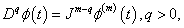where q is the order of fractional derivative,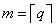, i.e., m is the first integer which is not less than q,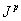is the p-order Riemann-Liouville fractional integral operator which is defined as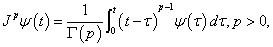where Γ(·) is the gamma function. In this work, the Caputo fractional derivative is employed. For the function h(t) having m-order continuous derivatives with t0, the Laplace transform of h(t) with the Caputo fractional derivative is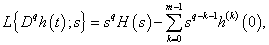where, q > 0, H(s) is the Laplace transform of the function h(t), and h(k)(0), k=0, 1, 2, …, m-1 are the initial conditions.

Consider a time-delayed fractional order drive system as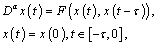(1)

where x(t) = (x1(t), x2(t), , xn(t))TRn is the state vector, α(0, 1) is the order of the fractional differential equation,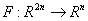is acontinuous function vector and τ>0 denotes the time-delay. Choose a time-delayed fractional order response system as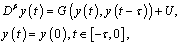(2)

where y(t) = (y1(t), y2(t), , yn(t))TRn is the state vector, β(0, 1) is the order of the fractional differential equation,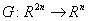is a continuous function vector, and U = (u1, u2, , un)T is a controller to be determined later. Without loss of  generality, decompose the response system (2) as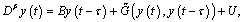(3)

where B=diag{b1, b2,…, bn}, biR+, i=1, 2,…, n is a given matrix,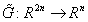is the corresponding remainder nonlinear  function vector.

Define the error state vector between systems (1) and (2) as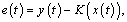(4)

where e(t)=(e1(t), e2(t), …, en(t))TRn, K(x(t))=(k1(x(t)), k2(x(t)),…, kn(x(t)))T is a continuous function vector. Then, e(t-τ)=y(t-τ)-K(x(t-τ)).

Definition 1 For the time-delayed fractional order drive system (1) and response system (2), it is said to be generalized synchronization if there exists a controller U such that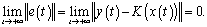(5)

Remark 1 If K(x(t))=(x1(t), x2(t), …, xn(t))T or K(x(t))=(-x1(t), -x2(t), …, -xn(t))T, the generalized synchronization is respectively simplified to the complete synchronization and the anti-phase synchronization. If K(x(t))=(k1x1(t), k2x2(t), …, knxn(t))T, kiR, i=1, 2, …, n, the generalized synchronization is reduced to the generalized projective synchronization. And if the function vector K(x(t))=(k1(x(t))x1(t), k2(x(t))x2(t), …, kn(x(t))xn(t))T, the generalized synchronization is considered as the function projective synchronization .

Remark 2 Both of systems with identical and different fractional orders can be applied to the generalized synchronization because the orders of the fractional derivative α and β may be different.

Remark 3 If the function vectors F(x(t), x(t-τ)) =G(y(t), y(t-τ)), the generalized synchronization between systems (1) and (2) is regarded as the synchronization of two identical time-delayed fractional order chaotic system with different initial conditions.

Remark 4 According to the idea of tracking control, K(x(t)) in the error state vector is a reference signal in order to achieve the goal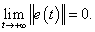Then, the generalized synchronization between systems (1) and (2) belongs to the problem of tracking control, i.e. the output signal y(t) follows the reference signal K(x(t)) ultimately.

Remark 5 If the time-delay τ=0, the generalized synchronization of time-delayed fractional order chaotic systems is changed into the synchronization of systems without time-delay. Compared with the synchronization of fractional order chaotic systems without time-delay, the generalized synchronization of time-delayed fractional order chaotic systems could get more secure communication in its applications to secure communication because of the unpredictability of the function vector K(x(t)), the time-delay τ and the fractional orders α, β.

With the parameters given above, a nonlinear controller is chosen as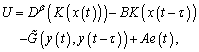(6)

where A = diag{a1, a2, …, an}, ARn×n is a feedback gain matrix to be designed later. Substituting the controller (6) into system (3), the error system is written as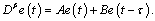(7)

Then, the generalized synchronization between systems (1) and (2) is transformed into the discussion of the asymptotical stability of the zero solution of system (7).

In Ref. , Deng et al. discussed the stability of an n-dimensional linear fractional differential system with multiple time-delays:(8)

where z(t) = (z1(t), z2(t), , zn(t))T is the state vector, qi (0, 1) is the order of the fractional derivative,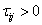is the time-delay, the initial values zi(t) = fi(t) are given for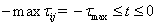, i, j=1, 2,…, n, and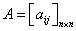is the coefficient matrix. Applying the Laplace transform to system (8), we obtain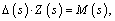where Z(s) = (Z1(s), Z2(s), , Zn(s))Tis the Laplace transform of state vector z(t) = (z1(t), z2(t), , zn(t))T, M(s) = (m1(s), m2(s), , mn(s))Tis the nonlinear part, and the characteristic matrixof system (8) isTheorem 1  If all the roots of the characteristic equation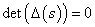have negative real parts, then the zero solution of system (8) is Lyapunov globally asymptotically stable.

Corollary 1  If q1=q2 = = qn = ρ (0, 1), all the eigenvalues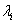, i = 1, 2, …, n of the coefficient matrix A satisfy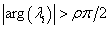, and the characteristic equationhas no purely imaginary roots for any, i, j=1, 2,…, n, then the zero solution of system (8) is Lyapunov globally asymptotically stable.

Then, a sufficient condition for the generalized synchronization between systems (1) and (2) can be obtained based on Corollary 1.

Theorem 2 For the time-delayed fractional order drive system (1) and response system (2), the generalized synchronization can be achieved if there exists a matrix A = diag{a1, a2, , an}Rn×n in controller (6) such that ai<-bi/sin(βπ/2), i=1, 2, …, n.

Proof. For the time-delayed fractional order error system (7), C = A+B is the coefficient matrix. The eigenvalues of the matrix C are λi=ai+bi< 0, i=1, 2, …, n due to the given conditions ai<-bi/sin(βπ/2), bi> 0, β(0, 1). Therefore, all the eigenvalues λi of the coefficient matrix C satisfy |arg(λi)| >π/2> βπ/2.

Taking Laplace transform on both sides of system (7) gives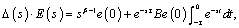where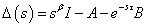is the characteristic matrix of system (7), E(s) is the Laplace transform of the error state vector e(t). The characteristic equation of system (7) is(9)

Suppose that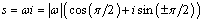is the root of the following equation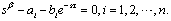(10)

Then, we have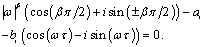Separating the real and imaginary parts, one can get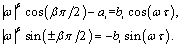Hence,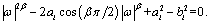(11)

For the given conditions ai<-bi/sin(βπ/2), bi> 0, β(0, 1), we can derive that the discriminant of the roots satisfies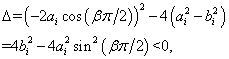which means that Eq.(11) has no real solutions. Thus, Eq. (9) has nopurely imaginary roots.

According to Corollary 1, the zero solution of the time-delayed fractional order error system (7) is globally asymptotically stable. And the generalized synchronization of time-delayed fractional order chaotic systems (1) and (2) is realized.

Remark 6 For the time-delayed systems, the current state vector of systems depends on the previous state vector. Introduction of delay in system enriches its dynamics and allows a precise description of the real life phenomena. Then, the discussion of synchronization for time-delayed systems is important and useful.

3. Numerical Simulations

Both identical and different structural time-delayed fractional order chaotic systems are applied for the generalized synchronization. And the approximate numerical solutions of the time-delayed fractional order differential equations are obtained based on the predictor-corrector scheme 

3.1. Synchronization of Time-Delayed Fractional Order Financial Systems

The generalized synchronization of two identical time-delayed fractional order chaotic financial systems  with different initial conditions is considered. The drive system is described by(12)

where x(t)=(x1(t), x2(t), x3(t))T is the statevector, α(0, 1) is the fractional order of system (12), a, b,c are the real positive parameters, τ>0 denotes the time-delay. When α=0.94, τ=0.05, (a, b, c)=(3, 0.1,1) and x(0)=(0.1, 4,0.5)T, the chaotic attractor of the time-delayed fractional order financial system (12) is shown in figure 1.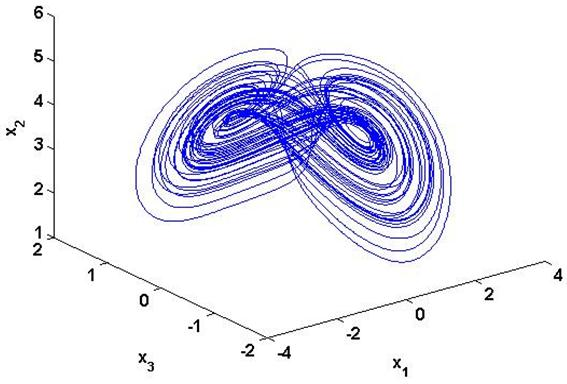Figure 1. The chaotic attractor of time-delayed fractional order financial system (12) with α=0.94, τ=0.05, (a, b, c)=(3, 0.1,1) and x(0)=(0.1, 4, 0.5)T.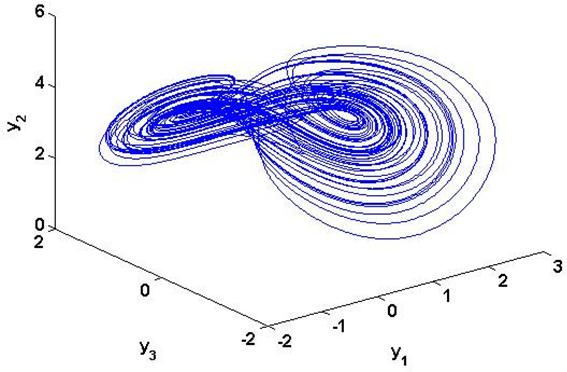Figure 2. The chaotic attractor of time-delayed fractional order financial system (13) with β=0.95, τ=0.05, (a, b,c)=(3, 0.1, 1) and y(0)=(0.5,2,1.5)T.

The response system is written as(13)

where y(t)=(y1(t), y2(t), y3(t))T is the statevector, β(0, 1) is the fractional order of system (13),U=(u1,u2,u3)T is the controller to be designed later. For β=0.95, τ=0.05, (a, b,c)=(3, 0.1, 1) and y(0)=(0.5,2,1.5)T, the chaotic attractor of the time-delayed fractional order financial system (13) is shown in figure 2. Based on the proposed method, the response system (13) can be rewritten as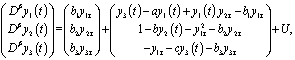(14)

where yiτ=yi(t-τ), i =1, 2, 3 are the simple notations, and B=diag{b1, b2,b3} is a given matrix which satisfies biR+, i=1, 2, 3.

The error state vector between systems (12) and (13) is defined as e(t)=y(t)-K(x(t)), where e(t)=(e1(t),e2(t),e3(t))T, K(x(t))=(k1(x(t)), k2(x(t)), k3(x(t)))T is a continuous function vector. Then, e(t-τ)=y(t-τ)-K(x(t-τ)).

Combining the proposed controller (6) and system (14), the error system is shown as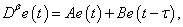where A=diag{α1, α2, α3} is a matrix to be determined later. Selecting ai<-bi/sin(βπ/2), i=1, 2, 3, the generalized synchronization of the time-delayed fractional order chaotic financial systems (12)-(13) is achieved based on Theorem 2.

For example, when α=0.94, β=0.95,τ=0.05, (a, b, c)=(3, 0.1,1), x(0)=(0.1, 4, 0.5)T and y(0)=(0.5, 2, 1.5)T, the drive and response systems (12)-(13) are chaotic. Setting K(x(t))=(15x1+sin(x2), -2x1x3, -0.5x2-x3)T and B=diag{1, 2, 3}, the generalized synchronization between systems (12) and (13) can be realized with A=diag{-2, -3, -5}. The phase diagrams of systems (12) and (13) are plotted together in figure 3(a). For displaying clearly, the phase diagram of system (13) is moved along the positive direction of the coordinate. The corresponding error state curves are shown in figure 3(b), which indicate the generalized synchronization between systems (12) and (13) is successfully achieved.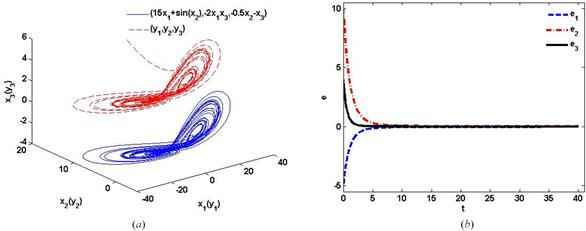Figure 3. The generalized synchronization between systems (12) and (13) with K(x(t))=(15x1+sin(x2), -2x1x3, -0.5x2-x3)T, A=diag{-2, -3, -5}, B=diag{1, 2, 3}: (a) the system attractors, (b) the error state curves.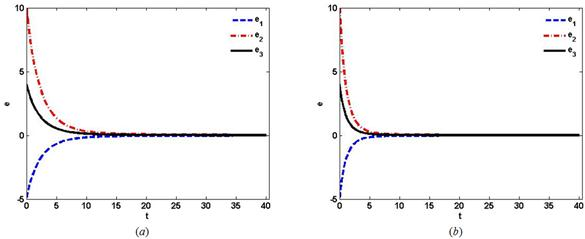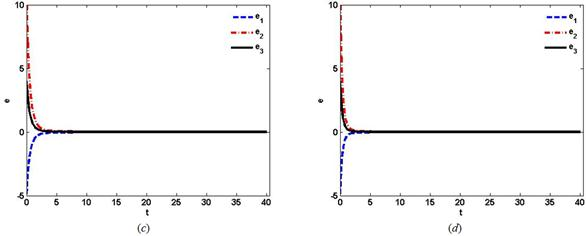Figure 4. The error state curves of the generalized synchronization between systems (12) and (13) with K(x(t))=(15x1+sin(x2), -2x1x3, -0.5x2-x3)T, B=diag{2, 2, 2} and(a) A =diag{-2.5, -2.5, -2.5}, (b) A =diag{-3, -3, -3}, (c) A =diag{-4, -4, -4}, (d) A =diag{-5, -5, -5}.

Due to Theorem 2, the generalized synchronization between systems (12) and (13) can be achieved if the matrix A = diag{a1, a2, a3} is subject to ai<-bi/sin(βπ/2), i = 1, 2, 3. Setting the matrix B = diag{2, 2, 2}, the error state curves are respectively shown in figures 4(a)-(d) with ai = -2.5, ai = -3, ai = -4 and ai = -5, i = 1, 2, 3, which indicate that the speed of the generalized synchronization can be increased via choosing the smaller values of ai, i = 1, 2, 3.

3.2. Synchronization Between Time-Delayed Fractional Order Liu System and Financial System

It is assumed that the time-delayed fractional order financial system drives the time-delayed fractional order Liu system . The drive system is written as (12). Whenα=0.94, τ=0.01, (a, b, c)=(3, 0.1, 1) and x(0)=(0.1, 4, 0.5)T, the chaotic attractor of system (12) is shown in figure 5. The response system is described by(15)

where y(t)=(y1(t), y2(t), y3(t))Tis the state vector, β(0, 1) is the fractional order of system (15), θ, η, γ are the real positive parameters, U=(u1, u2,u3)T is the controller to be designed later. For β=0.92, τ=0.01, (θ,η,γ)=(10, 40, 2.5) and y(0)=(2.2, 2.4, 38)T, thechaotic attractor of the time-delayed fractional order Liu system (15) is displayed in figure 6. According to the scheme mentioned above, the response system (15) can be rewritten as(16)

where yiτ=yi(t-τ), i =1, 2, 3 are the simple notations, and the given matrix B=diag{b1, b2,b3} satisfies biR+, i=1, 2, 3.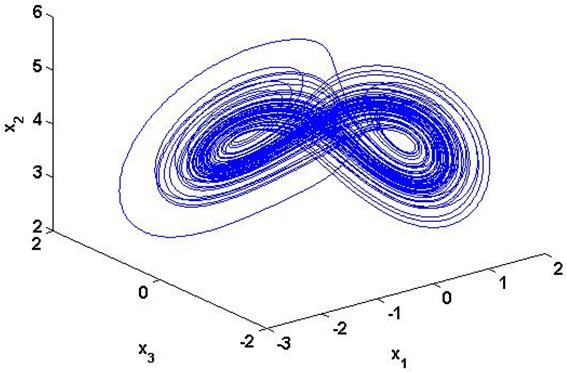Figure 5. The chaotic attractor of time-delayed fractional order financial system (12) with α=0.94, τ=0.01, (α, b, c)=(3, 0.1, 1) and x(0)=(0.1, 4, 0.5)T.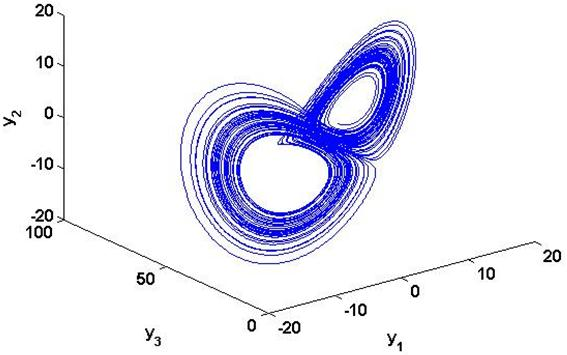Figure 6. The chaotic attractor of time-delayed fractional order Liu system (15) with β=0.92,τ=0.01, (θ, η,γ)= (10, 40, 2.5) and y(0)=(2.2,2.4, 38)T.

The error state vector between systems (12) and (15) is considered as e(t)=y(t)- K(x(t)), where e(t)=(e1(t),e2(t),e3(t))T, K(x(t))=(k1(x(t)), k2(x(t)), k3(x(t)))T is a continuous function vector. Then, e(t-τ)=y(t-τ)-K(x(t-τ)).

Substituting the controller (6) into system (16), the error system is obtained aswhere A =diag{α1, α2, α3} is a matrix to be determined later. Choosing ai<-bi/sin(βπ/2), i=1, 2, 3, the generalized synchronization between the time-delayed fractional order chaotic financial system (12) and Liu system (15) is realized based on Theorem 2.

For example, when α=0.94, β=0.92,τ=0.01,(a, b, c)=(3, 0.1, 1), (θ,η,γ)=(10, 40, 2.5), x(0)=(0.1, 4, 0.5)T and y(0)=(2.2, 2.4, 38)T, the drive and response systems (12) and (15) are chaotic. Setting K(x(t))=(-15x1-x2, 3x2+x3,10x2x3)T and B=diag{2, 3, 4}, the generalized synchronization between systems (12) and (15) can be achieved with A=diag{-3, -4.5, -5}. The phase diagrams of systems (12) and (15) are plotted together in figure 7(a). For displaying clearly, the phase diagram of system (15) is moved along the positive direction of the coordinate. The corresponding error state curves are displayed in figure 7(b), which indicate the generalized synchronization between systems (12) and (15) is successfully realized.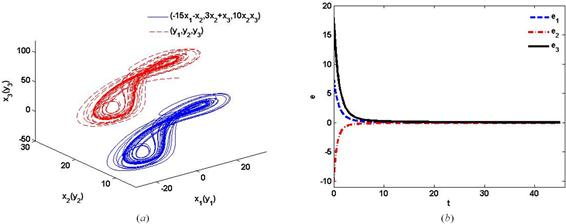Figure 7. The generalized synchronization between systems (12) and (15) with K(x(t))=(15x1-x2, 3x2+x3, 10x2x3)T, A=diag{-3, -4.5, -5}, B=diag{2, 3, 4}: (a) the system attractors, (b) the error state curves.

It is well-known that the system dynamics are always exposed to the external noise in practice. Then, the generalized synchronization between the time-delayed fractional order financial and Liu systems with bounded noise is considered. The time-delayed chaotic systems (12) and (15) affected by bounded noise can be rewritten as(17)

and(18)

whereare bounded noises of systems (12) and (15), respectively.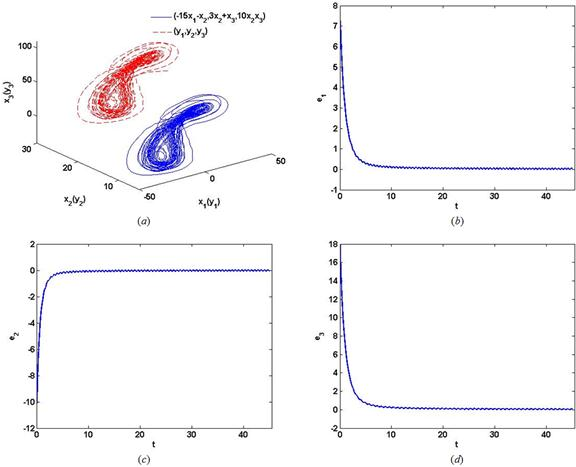Figure 8. The generalized synchronization between systems (17) and (18), and (a) the system attractors, (b)-(d) the error state curves.

Choose the system parameters and the initial conditions as before. The chaotic systems (17) and (18) realize the generalized synchronization based on the proposed synchronization scheme. figure 8(a) displays the phase diagrams of systems (17) and (18). And figure 8(b)-(d) show that the error states ei(t), i=1, 2, 3 converge to the relatively small intervals around zero. The time-delayed fractional order chaotic systems with bounded noise achieve the generalized synchronization in some sense. The proposed synchronization strategy has robustness against the effect of external noise.

4. Conclusions

A definition of generalized synchronization for fractional order chaotic systems with time-delays is given in this paper. Both complete synchronization and projective synchronization are the special cases of the generalized synchronization. On the basis of the stability theorem of linear time-delayed fractional order chaotic systems, a nonlinear fractional order controller is proposed for the synchronization of systems with identical and different structures. Finally, the time-delayed fractional order financial system and Liu system are applied to realize the synchronization. The synchronization speed can be improved via selecting an appropriate matrix A. And the controller is robust to the external bounded noise disturbances.

In the real applications, chaos synchronization is usually destroyed by external noise and system uncertainties. Then, the robust synchronization and the quasi-synchronization of time-delayed fractional order chaotic systems with unknown parameters are the interesting and significant problems for future study.

Acknowledgment

This paper is funnded by Beijing Key Laboratory (NO: BZ0211) and Beijing Intelligent Logistics System Collaborative Innovation Center.

References

1. Guanrong, Chen, Xiaoning Dong, From Chaos to Order: Methodologies, Perspectives and Applications, World Scientific, Singapore, 1998.
2. Louis M. Pecora, Thomas L. Carroll. Synchronization in chaotic systems. Physical Review Letters, 1990, 64(8):821-824.
3. Louis M. Pecora, Thomas L. Carroll. Driving systems with chaotic signals. Physical Review A, 1991, 44(4):2374-2383.
4. Chenggui Yao, Qi Zhao, Jun Yu. Complete synchronization induced by disorder in coupled chaotic lattices. Physics Letters A, 2013, 377(5):370-377.
5. Fangfei Li, Xiwen Lu. Complete synchronization of temporal Boolean networks. Neural Networks, 2013, 44:72-77.
6. Ronnie Mainieri, Jan Rehacek. Projective synchronization in three-dimensional chaotic systems. Physical Review Letters, 1999, 82(15):3024-3045.
7. C.Y. Chee, D. Xu. Chaos-based M-nary digital communication technique using controller projective synchronization. IEE Proceedings. G, Circuit, Devices and Systems, 2006, 153(4):357-360.
8. Nikolai F. Rulkov, Mikhail M. Sushchik, Lev S. Tsimring, Henry D. I. Abarbanel. Generalized synchronization of chaos in directionally coupled chaotic sytems. Physical Review E, 1995, 51(2):980-994.
9. Gerhard Keller, Haider H. Jafri, Ram Ramaswamy. Nature of weak generalized synchronization in chaotically driven maps. Physical Review E, 2013, 87(4):042913.
10. Aihua Hu, Zhenyuan Xu. Multi-stable chaotic attractors in generalized synchronization. Communications in Nonlinear Science and Numerical Simulation, 2011, 16(8):3237-3244.
11. Tanmoy Banerjee, Debabrata Biswas, B. C. Sarkar. Complete and generalized synchronization of chaos and hyperchaos in a coupled first-order time-delayed system. Nonlinear Dynamics, 2013, 71(1-2):279-290.
12. Xing He, Chuandong Li, Junjian Huang, Li Xiao. Generalized synchronization of arbitrary-dimensional chaotic systems. Optik-International Journal for Light and Electron Optics. 2015, 126(4):454-459.
13. Igor Podlubny, Fractional Differential Equations, Academic Press, New York, 1999.
14. Stefan G. Samko, Anatoly A. Kilbas, Qleg I. Marichev, Fractional Integrals and Derivatives: Theory and Applications, Gordon and Breach, New York, 1993.
15. R. Hilfer, Applications of Fractional Calculus in Physics, World Scientific, New Jersey, 2001.
16. Denis Matignon. Stability Results of Fractional Differential Equations with Applications to Control Processing. IMACS, IEEE-SMC, Lille, France, 1996.
17. Arman Kiani-B, Kia Fallahi, Naser Pariz, Henry Leung. A chaotic secure communication scheme using fractional chaotic systems based on an extended fractional Kalman filter. Communications in Nonlinear Science and Numerical Simulation, 2009, 14(3):863-879.
18. Ling Liu, Deliang Liang, Chongxin Liu, Qun Zhang. Nonlinear state observer design for projective synchronization of fractional-order permanent magnet synchronous motor. International Journal of Modern Physics B, 2012, 26(30):1250166.
19. Gangquan Si, Zhiyong Sun, Yanbin Zhang, Wenquan Chen.Projective synchronization of different fractional-order chaotic systems with non-identical orders. Nonlinear Analysis: Real World Applications, 2012, 13(4):1761-1771.
20. Kuntanapreeda Suwat. Robust synchronization of fractional-order unified chaotic systems via linear control. Computers &Mathematics with Applications, 2012, 63(1):183-190.
21. Dongfeng Wang, Jinying Zhang, Xiaoyan Wang. Synchronization of uncertain fractional-order chaotic systems with disturbance based on fractional terminal sliding mode controller. Chinese Physics B, 2013, 22(4):040507.
22. Mohammad Pourmahmood Aghababa. Finite-time chaos control and synchronization of fractional-order nonautonomous chaotic (hyperchaotic) systems using fractional nonsingular terminal sliding mode technique. Nonlinear Dynamics, 2012, 69(1-2):247-261.
23. Haiyan Hu, Zaihua Wang, Dynamics of Controlled Mechanical Systems with Delayed Feedback, Springer, Germany, 2002.
24. T.D. Frank. Time-dependent solutions for stochastic systems with delays: perturbation theory and applications to financial physics. Physics Letters A, 2006, 357(4-5):275-283.
25. Kunal Chakraborty, Milon Chakraborty, T. K. Kar. Bifurcation and control of a bioeconomic model of a prey-predator systems with a time-delay. Nonlinear Analysis: Hybrid Systems, 2011, 5(4):613-625.
26. Michael C. Mackey, Leon Glass. Oscillation and Chaos in Physiological Control Systems. Science, New Series, 1977, 197(4300):287-289.
27. Feng-Hsiag Hsiao. Optimal exponential synchronization of chaotic systems with multiple time delays via fuzzy control. Abstract and Applied Analysis, 2013, 742821.
28. Abdujelil Abdurahman, Haijun Jiang, Zhidong Teng. Finite-time synchronization for memristor-based neural networks with time-varing delays. Neural Networks, 2015, 69:20-28.
29. Lixiang Li, Haipeng Peng, Yixian Yang, Xiangdong Wang. On the chaotic synchronization of Lorenz systems with time-varying lags. Chaos Solitons & Fractals, 2009, 41(2):783-794.
30. Thongchai Botmart, Piyapong Niamsup, X. Liu. Synchronization of non-autonomous chaotic systems with time-varying delay via delayed feedback control. Communications in Nonlinear Science and Numerical Simulation, 2012, 17(4):1894-1907.
31. Chuandong Li, Xiaofeng Liao, Kwok-wo Wong. Chaotic lag synchronization of coupled time-delayed systems and its applications in secure communication. Physica D: Nonlinear Phenomena, 2004, 194(3-4):187-202.
32. Weihua Deng, Changpin Li, Jinhu Lü. Stability analysis of linear fractional differential system with multiple time delays. Nonlinear Dynamics, 2007, 48:409-416.
33. Shangbo Zhou, Xiaoran Lin, Hua Li. Chaotic synchronization of a fractional-order systems based on washout filter control. Communications in Nonlinear Science and Numerical Simulation, 2011, 16(3):1533-1540.
34. Reza Behinfaraz, Mohammad Ali Badamchizadeh, Amir Rikhtegar Ghiasi. An approach to achieve modified projective synchronization between different types of fractional-order chaotic systems with time-varying delays. Chaos, Solitons & Fractals, 2015, 78:95-106.
35. Ping Zhou, Wei Zhu. Function projective synchronization for fractional-order chaotic systems. Nonlinear Analysis: Real World Applications, 2011, 12(2):811-816.
36. Sachin Bhalekar, VarshaDaftardar-Gejji. A predictor-corrector scheme for solving nonlinear delay differential equations of fractional order. Journal of Fractional Calculus and Applications, 2011, 1(5):1-8.
37. Zhen Wang, Xia Huang, Guodong Shi. Analysis of nonlinear dynamics and chaos in a fractional order financial system with time delay. Computers and Mathematics with Applications, 2011, 62(3):1531-1539.
38. Sachin Bhalekar, Varsha Daftardar-Gejji. Fractional ordered Liu system with time-delay. Communications in Nonlinear Science and Numerical Simulation, 2010, 15(8):2178-2191.

 Contents 1. 2. 3. 3.1. 3.2. 4.
Article ToolsAbstractPDF(518K)
Follow on us
PUBLICATION SERVICE
JOIN US
RESOURCES
SPECIAL SERVICES
ADDRESS
Science Publishing Group
548 FASHION AVENUE
NEW YORK, NY 10018
U.S.A.
Tel: (001)347-688-8931